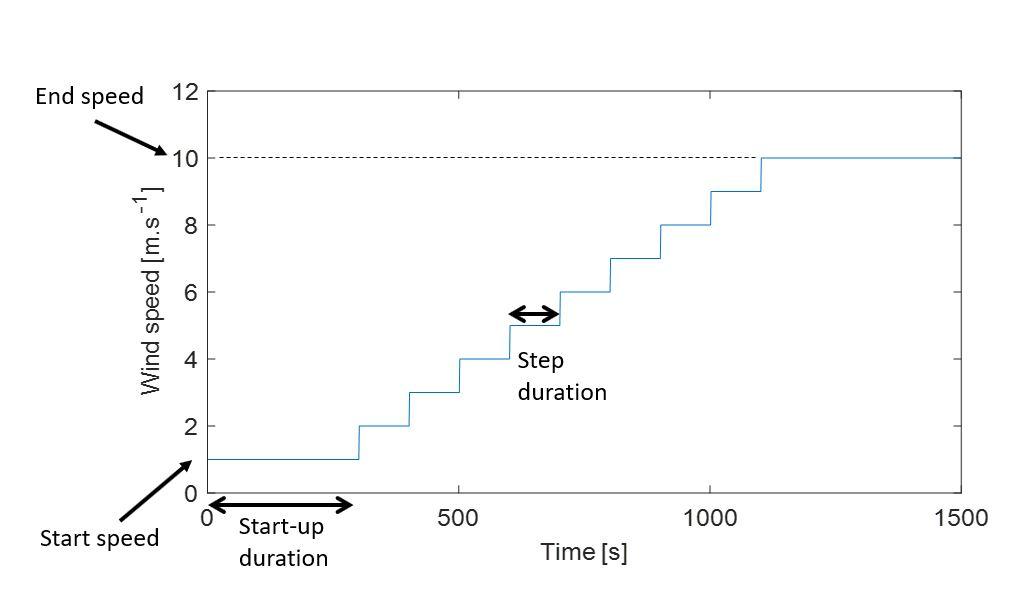# Stepwise wind

The Stepwise wind can be defined from parameters or selected from file. The parameters in the Properties pane enable you to define it from parameters. The parameters for the stepwise wind are illustrated in the following figure. The vertical step for the wind speed is defined by the number of steps (in th example shown below, the number of steps is equal to 9).

If you want to use your own wind history, you can define a Stepwise wind from file and use it as the input for the simulation. In that case, you can define the variation of the wind speed over time (see Stepwise wind file).

The equations defining the wind direction and wind angle for parameter based or imported stepwise wind can be found in the Stepwise Wind section of the Theory manual.## Stepwise

### Start speed

The start (initial) speed.

For more information on how the stepwise wind is computed, check the StepWise wind section of the theory manual.

• Default value: 3
• Unit: ${\text{m}} \over {\text{s}}$
• Range: -100 — 100

### End speed

The end speed.

• Default value: 25
• Unit: ${\text{m}} \over {\text{s}}$
• Range: -100 — 100

### Start angle

The start angle.
Min: -360°, max: 360°

• Default value: 0
• Unit: $°$
• Range: -360 — 360

### End angle

The ending angle.
Min: -360°, max: 360°

• Default value: 0
• Unit: $°$
• Range: -360 — 360

### Start-up duration

Duration until first speed/angle change

• Default value: 10
• Unit: $\text{s}$
• Range: 0 — 1e+06

### Num. of steps

Number of steps with wind speed and angle change

• Default value: 22
• Unit:
• Range: 1 — 1e+06

### Step duration

Duration of each step.

• Default value: 5
• Unit: $\text{s}$
• Range: 0.1 — 1e+06

### Input file

The file containing the wind speeds and angles per time.

Note: this parameter is only accessible in the batch manager. In order to import auser-defined stepwise wind in time simulation, use the Action buttons at the top of the parameter window.

The format of the input file is expained in the Stepwise wind file section.

• Default value: Data/StepwiseWind/default.txt
• Unit:

## Shear profile

### Shear

Decides if a shear law is applied to the deterministic wind.

• Default value: False
• Unit:

### Power law exponent

The shear is computed using a power law. This is the power law exponent.

To see how the power law is computed, see Wind profile power law

• Default value: 0.2
• Unit:
• Range: 0 — 100

### Reference height scheme

This parameter lets your define whether the point of application of the reference speed (used to compute a vertical wind shear) is input by you or defined as the hub height. This parameter is only relevant if a shear law is applied to the wind.

IHub height is selected, the reference wind speed for the shear will be applied at hub height. If the turbine has multiple rotors, the reference wind speed will be applied at the average hub height. If there is no rotor, the reference speed is applied at the average height of the nodes at sea level.

Options:

Hub height (default):

The reference height is set to the hub height.

User defined:

The reference height is set explicitly by the user (you).

### Reference height

The height above the ground (or above sea level for offshore turbine) where the reference wind speed is applied.

Hub height is often used for the reference height, but in some cases it is convenient to use another height. E.g. if the wind speed is given for a height of 10 m above ground, then this parameter should be set to 10 m. Note: The reference height only has an influence if there is shear (see Wind profile power law)

• Default value: 10
• Unit: $\text{m}$
• Range: 0.01 — 1000